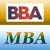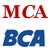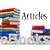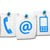# Primal Problem: Duality Example

Now I will show you an example of primal problem.

Minimize z = 3x1 + 3x2

subject to

2x1 + 4x2 ≥ 40
3x1 + 2x2 ≥ 50

x1, x2 ≥ 0

Solution.

We use the M method to solve this problem.
After adding surplus & artificial variables, we have

Minimize z = 3x1 + 3x2 + 0x3 + 0x4 + MA1 + MA2

2x1 + 4x2 - x3 + A1 = 40
3x1 + 2x2 - x4 + A2 = 50
x1, x2, x3, x4, A1, A2 ≥ 0

Where:
A1 and A2 are artificial variables.
x3 and x4 are surplus variables.

##### Initial basic feasible solution

x1 = 0, x2 = 0, x3 = 0, x4 = 0
A1 = 40, A2 = 50

#### Table 1: Simplex Method

On small screens, scroll horizontally to view full calculation

cj 3 3 0 0 M M
cB Basic variables
B
x1 x2 x3 x4 A1 A2 Solution values
b (=XB)
M A1 2 4 -1 0 1 0 40
M A2 3 2 0 -1 0 1 50
zj-cj   5M - 3 6M - 3 -M -M 0 0

Choose the highest positive value from index row.
As 6M > 5M, x2 becomes a basic variable in the next iteration.
Minimum (40/4, 50/2) = (10, 25) = 10
Key Row = A1 row.
Pivot element = 4.
A1 departs and x2 enters.

Table 2

cj 3 3 0 0 M
cB Basic variables
B
x1 x2 x3 x4 A2 Solution values
b (=XB)
3 x2 1/2 1 -1/4 0 0 10
M A2 2 0 1/2 -1 1 30
zj-cj   2M - 3/2 0 M/2 - 3/4 -M 0

Table 3

Use Horizontal Scrollbar to View Full Table Calculation

cj 3 3 0 0
cB Basic variables
B
x1 x2 x3 x4 Solution values
b (=XB)
3 x2 0 1 -3/8 1/4 5/2
3 x1 1 0 1/4 -1/2 15
zj-cj   0 0 -3/8 -3/4

Since all the values of zj-cj ≤ 0, therefore, the current solution is the optimal solution.

The optimal solution is

x1 = 15, x2 = 5/2
z = 3 X 15 + 3 X 5/2= 105/2.

Note down the values of zj-cj under the surplus variables x3 and x4 on a piece of paper.

Share and Recommend

##### Menu
•MBA & BBA
•BCA & MCA
•Ebooks & Articles
•Contact us